# Scientific Notation Scientific Notation 4 632 x Coefficient

• Slides: 16Scientific Notation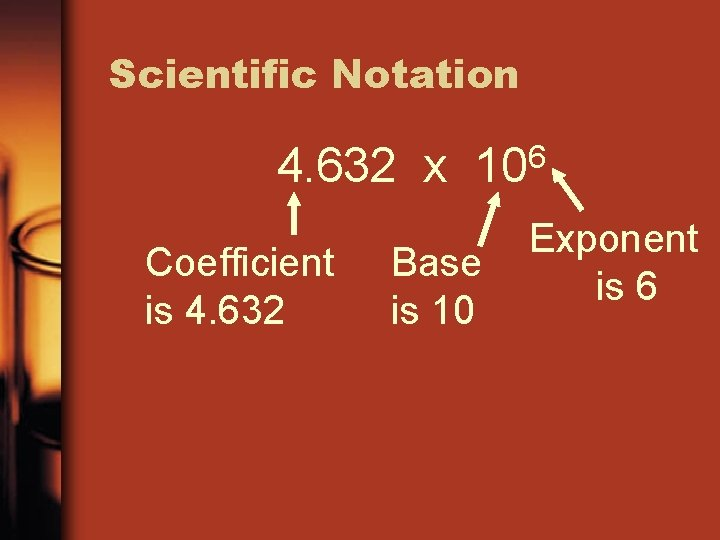Scientific Notation 4. 632 x Coefficient is 4. 632 6 10 Base is 10 Exponent is 6IMPORTANT RULES 4. 632 x 106 The coefficient is always larger than or equal to 1, and smaller than 10. The base is always 10. The exponent is positive for large numbers, and negative for numbers less than 1. The exponent can not be a decimal (like 3. 5)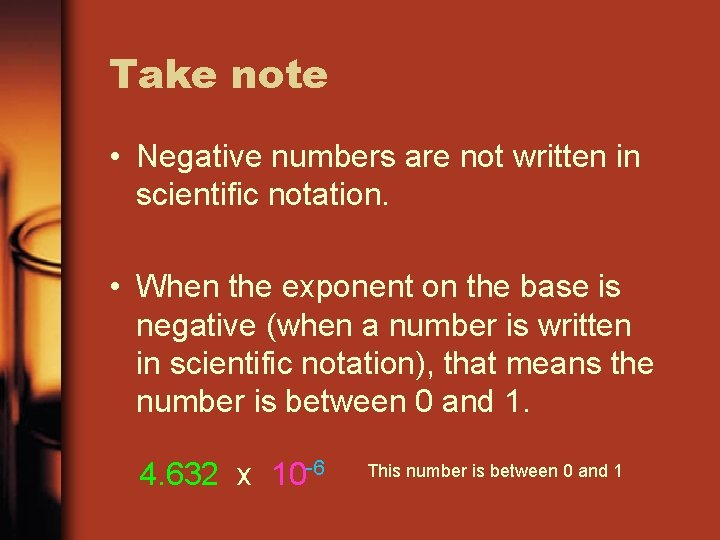Take note • Negative numbers are not written in scientific notation. • When the exponent on the base is negative (when a number is written in scientific notation), that means the number is between 0 and 1. 4. 632 x 10 -6 This number is between 0 and 1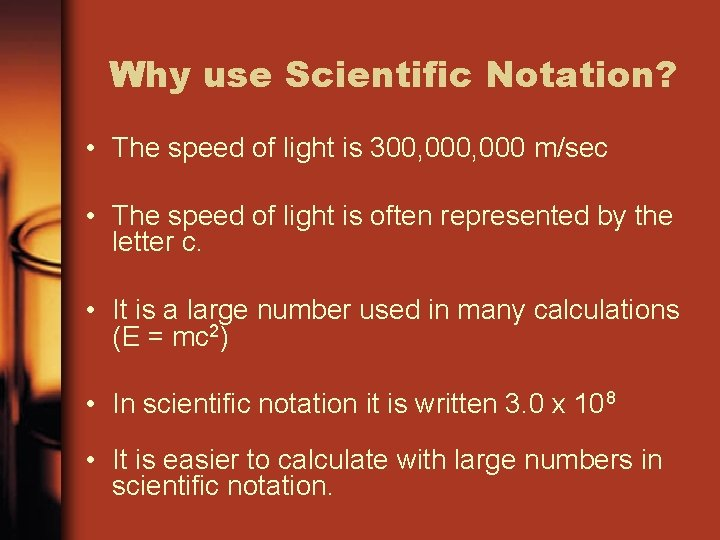Why use Scientific Notation? • The speed of light is 300, 000 m/sec • The speed of light is often represented by the letter c. • It is a large number used in many calculations (E = mc 2) • In scientific notation it is written 3. 0 x 108 • It is easier to calculate with large numbers in scientific notation.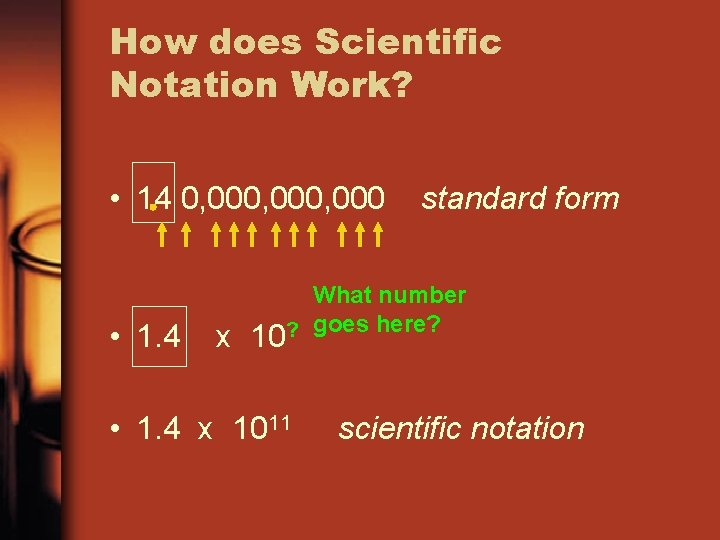How does Scientific Notation Work? • 14 0, 000, 000 • 1. 4 x standard form What number goes here? ? 10 • 1. 4 x 1011 scientific notationScientific Notation: Great for very small numbers • The size of a dust particle is about 0. 000 000 752 kilograms • 0. 000 000 752 • 7. 52 standard form x 10? • 7. 52 x 10 -10 scientific notationWhat does Scientific Notation look like on the HP 39 G ? Find the EEX key To access these second functions, you need to hit the SHIFT key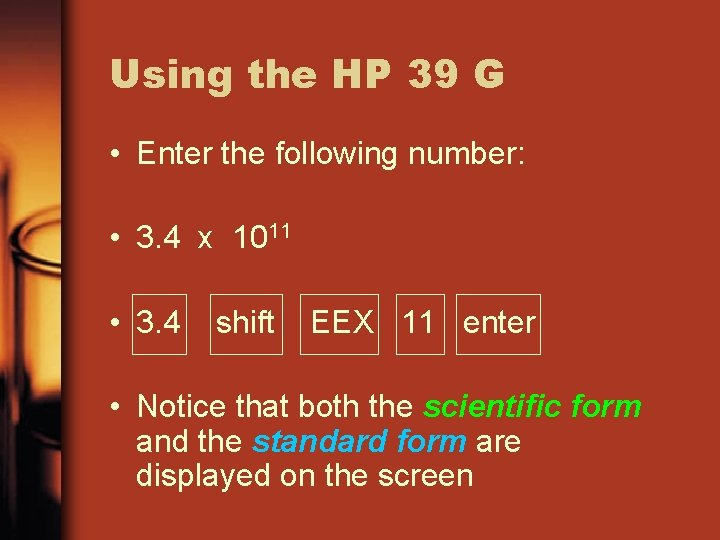Using the HP 39 G • Enter the following number: • 3. 4 x 1011 • 3. 4 shift EEX 11 enter • Notice that both the scientific form and the standard form are displayed on the screen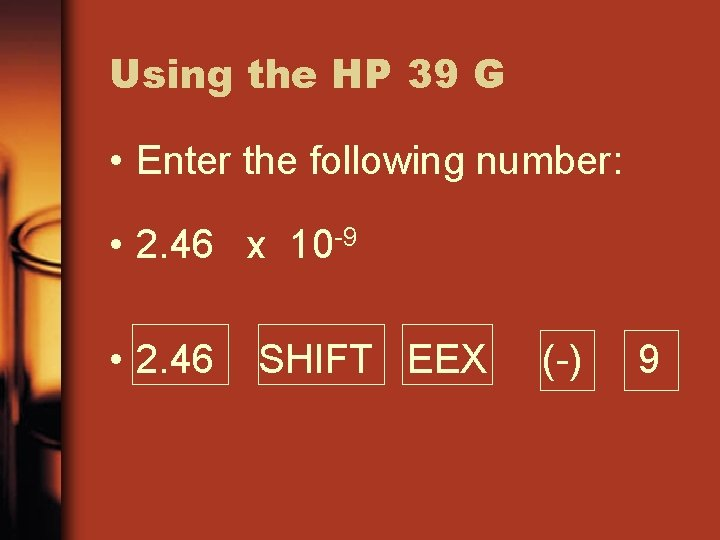Using the HP 39 G • Enter the following number: • 2. 46 x 10 -9 • 2. 46 SHIFT EEX (-) 9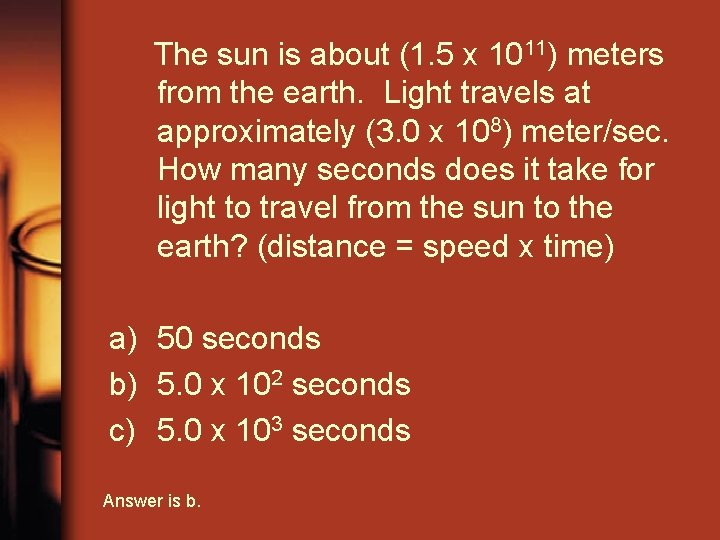The sun is about (1. 5 x 1011) meters from the earth. Light travels at approximately (3. 0 x 108) meter/sec. How many seconds does it take for light to travel from the sun to the earth? (distance = speed x time) a) 50 seconds b) 5. 0 x 102 seconds c) 5. 0 x 103 seconds Answer is b.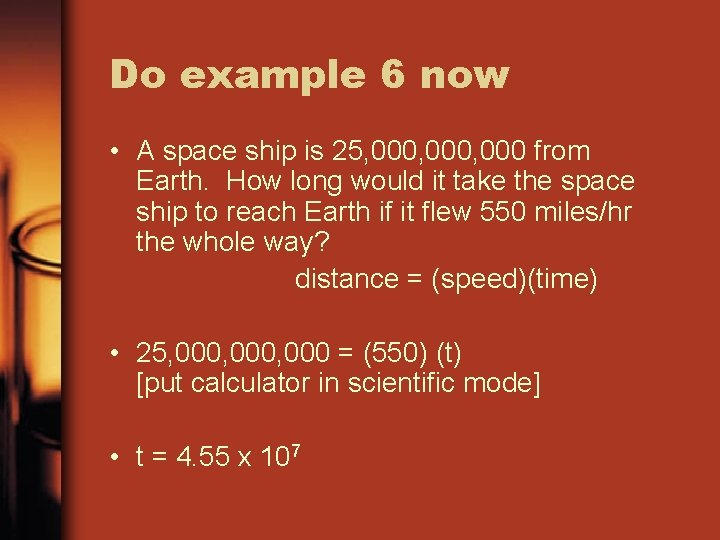Do example 6 now • A space ship is 25, 000, 000 from Earth. How long would it take the space ship to reach Earth if it flew 550 miles/hr the whole way? distance = (speed)(time) • 25, 000, 000 = (550) (t) [put calculator in scientific mode] • t = 4. 55 x 107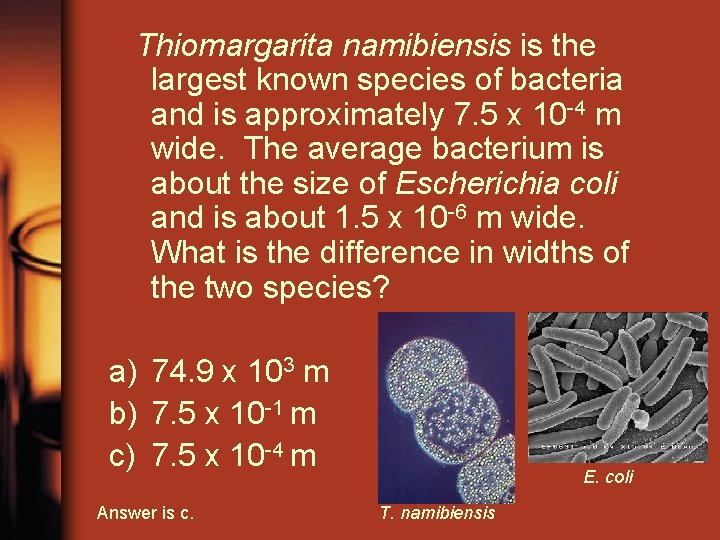Thiomargarita namibiensis is the largest known species of bacteria and is approximately 7. 5 x 10 -4 m wide. The average bacterium is about the size of Escherichia coli and is about 1. 5 x 10 -6 m wide. What is the difference in widths of the two species? a) 74. 9 x 103 m b) 7. 5 x 10 -1 m c) 7. 5 x 10 -4 m Answer is c. E. coli T. namibiensis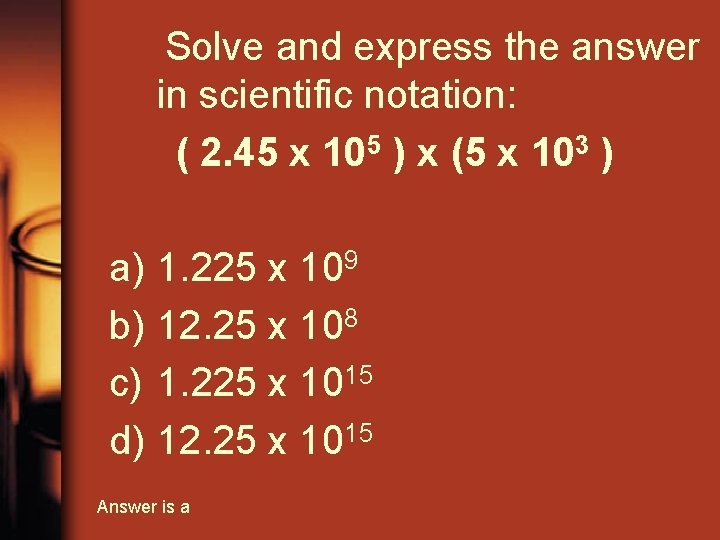Solve and express the answer in scientific notation: ( 2. 45 x 105 ) x (5 x 103 ) a) 1. 225 x 109 b) 12. 25 x 108 c) 1. 225 x 1015 d) 12. 25 x 1015 Answer is aDo example 5 now Multiply the following numbers. Express your answer in scientific notation. (3. 4 x 105) x ( 4. 8 x 107) Answer is 1. 632 x 1013 calculator emulatorDegree of accuracy • What is the degree of accuracy for the number 854. 4463 ? • The 3 is in the 10, 000 ths place so the degree of accuracy is 1/10, 000